GFG App
Open AppBrowser
Continue

# How to Group Pandas DataFrame By Date and Time ?

In this article, we will discuss how to group by a dataframe on the basis of date and time in Pandas. We will see the way to group a timeseries dataframe by Year, Month, days, etc. Additionally, we’ll also see the way to groupby time objects like minutes.

Pandas GroupBy allows us to specify a groupby instruction for an object. This specified instruction will select a column via the key parameter of the grouper function along with the level and/or axis parameters if given, a level of the index of the target object/column.

Syntax: pandas.Grouper(key=None, level=None, freq=None, axis=0, sort=False)

Below are some examples that depict how to group by a dataframe on the basis of date and time using pandas Grouper class.

Example 1: Group by month

## Python3

 `# importing modules ` `import` `pandas as pd ` ` `  `# creating a dataframe df ` `df ``=` `pd.DataFrame( ` `    ``{ ` `        ``"Date"``: [ ` `            ``pd.Timestamp(``"2000-11-02"``), ` `            ``pd.Timestamp(``"2000-01-02"``), ` `            ``pd.Timestamp(``"2000-01-09"``), ` `            ``pd.Timestamp(``"2000-03-11"``), ` `            ``pd.Timestamp(``"2000-01-26"``), ` `            ``pd.Timestamp(``"2000-02-16"``) ` `        ``], ` `        ``"ID"``: [``1``, ``2``, ``3``, ``4``, ``5``, ``6``], ` `        ``"Price"``: [``140``, ``120``, ``230``, ``40``, ``100``, ``450``] ` `    ``} ` `) ` ` `  `# show df ` `display(df) ` ` `  `# applying the groupby function on df ` `df.groupby(pd.Grouper(key``=``'Date'``, axis``=``0``,  ` `                      ``freq``=``'M'``)).``sum``() `

Output: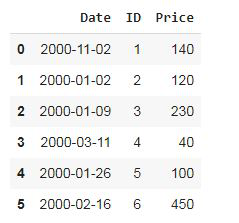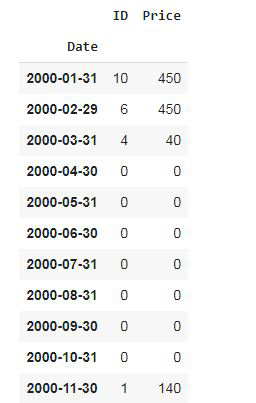In the above example, the dataframe is groupby by the Date column. As we have provided freq = ‘M’ which means month, so the data is grouped month-wise till the last date of every month and provided sum of price column. We have not provided value for all months, then also groupby function displayed data for all months and assigned value 0 for other months.

Example 2: Group by days

## Python3

 `# importing modules ` `import` `pandas as pd ` ` `  `# creating a dataframe df ` `df ``=` `pd.DataFrame( ` `    ``{ ` `        ``"Date"``: [ ` `            ``pd.Timestamp(``"2000-11-02"``), ` `            ``pd.Timestamp(``"2000-01-02"``), ` `            ``pd.Timestamp(``"2000-01-09"``), ` `            ``pd.Timestamp(``"2000-03-11"``), ` `            ``pd.Timestamp(``"2000-01-26"``), ` `            ``pd.Timestamp(``"2000-02-16"``) ` `        ``], ` `        ``"ID"``: [``1``, ``2``, ``3``, ``4``, ``5``, ``6``], ` `        ``"Price"``: [``140``, ``120``, ``230``, ``40``, ``100``, ``450``] ` `    ``} ` `) ` ` `  `# display dataframe ` `display(df) ` ` `  `# applying groupby ` `df.groupby(pd.Grouper(key``=``'Date'``, axis``=``0``,  ` `                      ``freq``=``'2D'``, sort``=``True``)).``sum``() `

Output: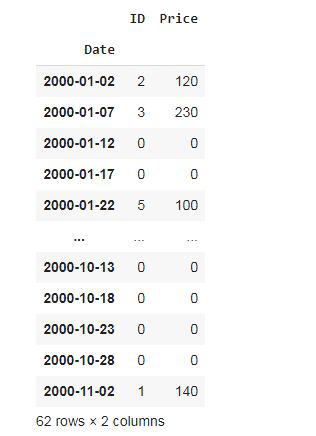In the above example, the dataframe is groupby by the Date column. As we have provided freq = ‘5D’ which means five days, so the data grouped by interval 5 days of every month till the last date given in the date column.

Example 3: Group by year

## Python3

 `# importing module ` `import` `pandas as pd ` ` `  `# creating dataframe with datetime ` `df ``=` `pd.DataFrame( ` `    ``{ ` `        ``"Date"``: [ ` ` `  `            ``# here the date contains ` `            ``# different years ` `            ``pd.Timestamp(``"2010-11-02"``), ` `            ``pd.Timestamp(``"2011-01-02"``), ` `            ``pd.Timestamp(``"2013-01-09"``), ` `            ``pd.Timestamp(``"2014-03-11"``), ` `            ``pd.Timestamp(``"2015-01-26"``), ` `            ``pd.Timestamp(``"2012-02-16"``) ` `        ``], ` `        ``"ID"``: [``1``, ``2``, ``3``, ``4``, ``5``, ``6``], ` `        ``"Price"``: [``140``, ``120``, ``230``, ``40``, ``100``, ``450``] ` `    ``} ` `) ` `# show df ` `display(df) ` ` `  `# applying groupby function ` `df.groupby(pd.Grouper(key``=``'Date'``, freq``=``'2Y'``)).``sum``() `

Output: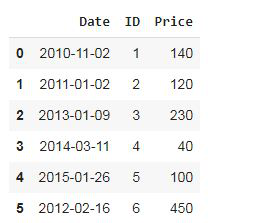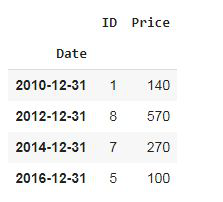In the above example, the dataframe is groupby by the Date column. As we have provided freq = ‘2Y’ which means 2 years, so the data is grouped in the interval of 2 years.

Example 4: Group by minutes

## Python3

 `# importing module ` `import` `pandas as pd ` ` `  `# create an array of 5 dates starting  ` `# at '2015-02-24', one per minute ` `dates ``=` `pd.date_range(``'2015-02-24'``, periods``=``10``, freq``=``'T'``) ` ` `  `# creating dataframe with above array  ` `# of dates ` `df ``=` `pd.DataFrame({``"Date"``: dates, ``"ID"``: [``1``, ``2``, ``3``, ``4``, ``5``, ``6``, ``7``, ``8``, ``9``, ``10``], ` `                   ``"Price"``: [``140``, ``120``, ``230``, ``40``, ``100``, ``450``, ``234``, ``785``, ``12``, ``42``]}) ` ` `  `# display dataframe ` `display(df) ` ` `  `# applied groupby function ` `df.groupby(pd.Grouper(key``=``'Date'``, freq``=``'2min'``)).``sum``() `

Output: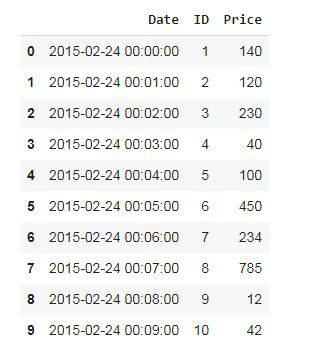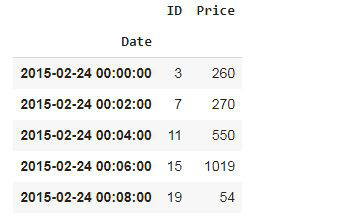In the above example, the data is grouped in intervals of every 2 minutes.

My Personal Notes arrow_drop_up